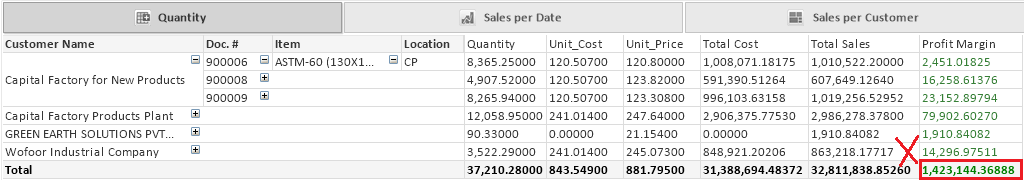# New to QlikView

Discussion board where members can get started with QlikView.

Contributor II

## Calculation in pivot table

why the calculation in pivot table is not accurate it give a wrong number, but in straight table it's right !!1 Solution

Accepted Solutions
Valued Contributor II

## Re: Calculation in pivot table

hi there,

It depends on what is the level of your aggrigation,

use the following exp

=sum(aggr(

((sum(QTY) + (vQty2)) * (sum([Unit Price])* (1+vSales2/100)))

-

(

((sum([Unit Cost]) * sum(QTY))) * (1+vCost2/100)

)

,

[Customer Name],

[SOP Number],

Item_Description,

[S_Location Code]

))

6 RepliesMVP

## Re: Calculation in pivot table

Provide sample application or sample data to work...

Contributor II

qlik example

Highlighted
Contributor II

## Re: Calculation in pivot table

I put it in last comment.

regards.MVP

## Re: Calculation in pivot table

There are so many information and objects in your qvw. We need to have only those two where you are trying to match!

Valued Contributor II

## Re: Calculation in pivot table

hi there,

It depends on what is the level of your aggrigation,

use the following exp

=sum(aggr(

((sum(QTY) + (vQty2)) * (sum([Unit Price])* (1+vSales2/100)))

-

(

((sum([Unit Cost]) * sum(QTY))) * (1+vCost2/100)

)

,

[Customer Name],

[SOP Number],

Item_Description,

[S_Location Code]

))

Contributor II

Thanks a lot.